# Applying Angle Theorems Projector Resources Applying Angle Theorems

• Slides: 9Applying Angle Theorems Projector Resources Applying Angle Theorems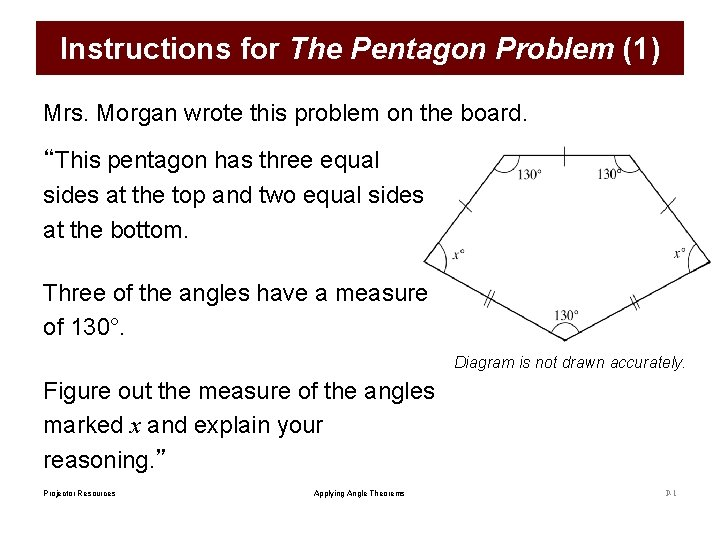Instructions for The Pentagon Problem (1) Mrs. Morgan wrote this problem on the board. “This pentagon has three equal sides at the top and two equal sides at the bottom. Three of the angles have a measure of 130°. Diagram is not drawn accurately. Figure out the measure of the angles marked x and explain your reasoning. ” Projector Resources Applying Angle Theorems P-1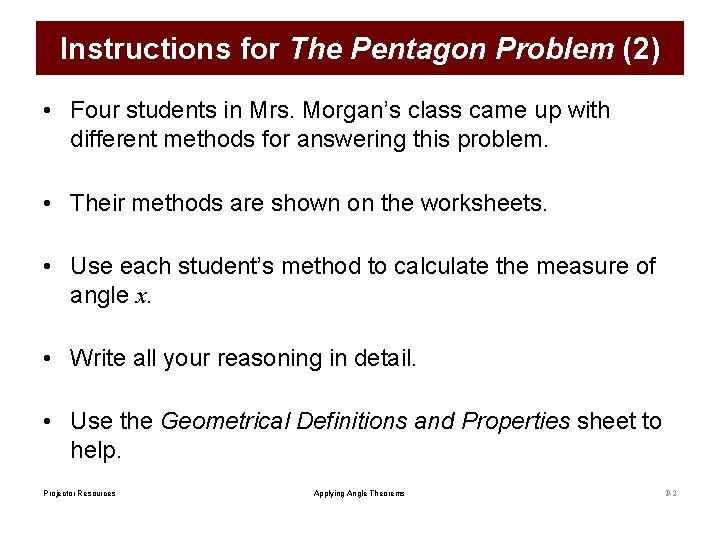Instructions for The Pentagon Problem (2) • Four students in Mrs. Morgan’s class came up with different methods for answering this problem. • Their methods are shown on the worksheets. • Use each student’s method to calculate the measure of angle x. • Write all your reasoning in detail. • Use the Geometrical Definitions and Properties sheet to help. Projector Resources Applying Angle Theorems P-2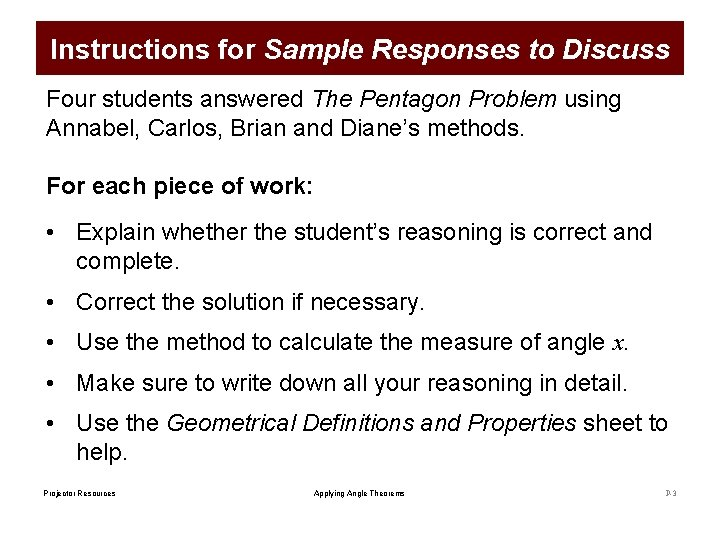Instructions for Sample Responses to Discuss Four students answered The Pentagon Problem using Annabel, Carlos, Brian and Diane’s methods. For each piece of work: • Explain whether the student’s reasoning is correct and complete. • Correct the solution if necessary. • Use the method to calculate the measure of angle x. • Make sure to write down all your reasoning in detail. • Use the Geometrical Definitions and Properties sheet to help. Projector Resources Applying Angle Theorems P-3Erasmus used Annabel’s method Projector Resources Applying Angle Theorems P-4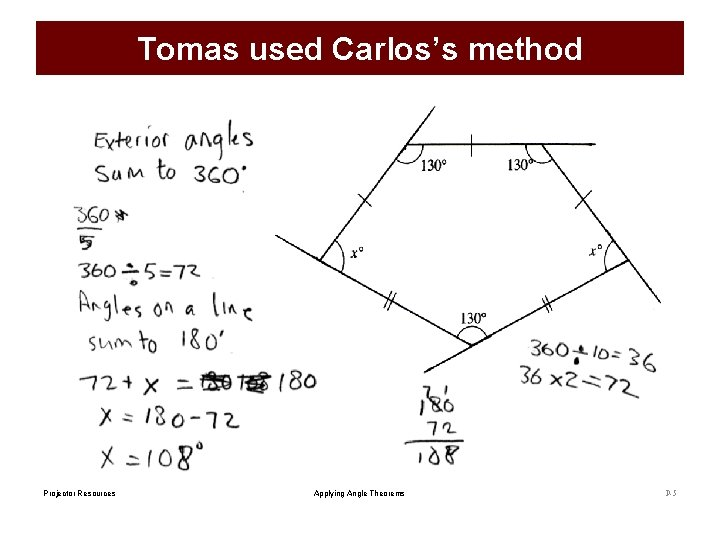Tomas used Carlos’s method Projector Resources Applying Angle Theorems P-5Katerina used Brian’s method Projector Resources Applying Angle Theorems P-6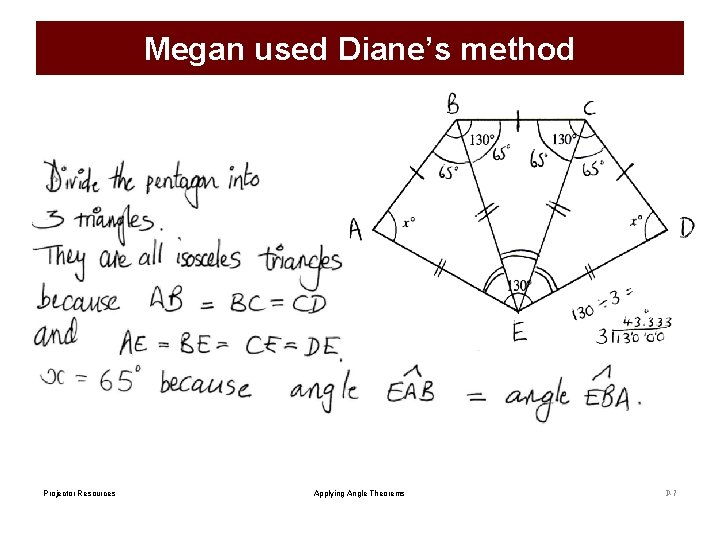Megan used Diane’s method Projector Resources Applying Angle Theorems P-7A semi-regular tessellation of pentagons and rhombuses Projector Resources Applying Angle Theorems P-8